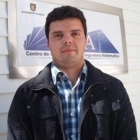﻿﻿ CI²MA - Publications | Graduate Thesis

# Graduate Thesis of Carlos GarcíaProgramPhD in Applied Sciences with mention in Mathematical Engineering, Universidad de Concepción
Enrollment Year2012
Senior Year2017
Thesis TitleMixed Finite Element Methods for Time-Dependent Wave Propagation Problems: Elastodynamics and Elastoacoustics

#### Thesis Summary:

This thesis aims at the formulation, analysis and implementation of mixed finite element methods for the time-dependent wave propagation models arising in elastodynamic and elastoacoustic. The analysis of the dependence on time of the formulations of these problems is one of the main goals of this thesis. This motivates us to follow the traditional approach for this kind of evolutive models, which consists of splitting the analysis into two steps: the first one deals with the semidiscrete scheme, in which only the space is discretized, whereas the second one refers to the fully-discrete scheme, where both space and time are discretized by using finite elements and finite differences, respectively. For the elastodynamic problem, the unknowns of our formulation are the stress and rotation tensors, where the latter is introduced as a Lagrange multiplier taking care of the symmetry of the stress tensor in a weak sense. Once the well-posedness of the formulation is established, we introduce a semidiscretization of the problem using a family of mixed finite elements satisfying standard hypotheses for the elasticity problem with reduced symmetry, and then prove the abstract error estimates. Finally, we use the Newmark method to obtain a fully-discrete version of our formulation and derive the corresponding error estimates as well. An interesting feature of our formulation is the fact that it does not involve the displacement since this is replaced in terms of the stress thanks to the momentum equation. Nevertheless, we show that this unknown can be easily recovered later on by means of a post-processing formula, thus leading to a suitable approximation of it. Moreover, a similar post-processing technique allows to obtain a numerical approximation of the acceleration. On the other hand, the unknowns of the formulation for the fluid-structure interaction problem are given by the stress tensor in the solid and the pressure in the fluid. Once the wellˆaposedness of the formulation is proved, we proceed to build an appropriate auxiliary operator whose properties are useful for the analysis of the semidiscrete scheme, and then we obtain the corresponding error estimates using the Arnold-Falk-Whinter mixed finite elements in the solid and the classic Lagrange elements in the fluid domain. The analysis of the corresponding fully-discrete scheme for this problem is performed in a separate chapter. Finally, we remark that for both problems analyzed in this thesis, we have shown that the discrete schemes employed are uniformly stable with respect to the discretization parameters, and have derived the respective asymptotic error estimates. In particular, we prove that they are immune to locking phenomenon in the nearly incompressible case. In addition, numerical experiments supporting the theoretical results and illustrating the robustness of each method are reported.

Thesis Director(s) Gabriel N. Gatica, Salim Meddahi
Thesis Project Approval Date2014, September 23
Thesis Defense Date2017, June 09
Professional MonitoringSince September 2017: postdoctorate at UBB under the supervision of Ricardo Oyarzua
PDF ThesisDownload Thesis PDF#### ISI Publications from the Thesis

Carlos GARCIA, Gabriel N. GATICA, Antonio MARQUEZ, Salim MEDDAHI: A fully discrete scheme for the pressure-stress formulation of the time-domain fluid-structure interaction problem. Calcolo, vol. 54, 4, pp. 1419-1439, (2017).

Carlos GARCIA, Gabriel N. GATICA, Salim MEDDAHI: Finite element semidiscretization of a pressure-stress formulation for the time-domain fluid-structure interaction problem. IMA Journal of Numerical Analysis, vol. 37, 4, pp. 1772-1799, (2017).

Carlos GARCIA, Gabriel N. GATICA, Salim MEDDAHI: A new mixed finite element method for elastodynamics with weak symmetry. Journal of Scientific Computing, vol. 72, 3, pp. 1049-1079, (2017).

Carlos GARCIA, Gabriel N. GATICA, Salim MEDDAHI: A new mixed finite element analysis of the elastodynamic equations. Applied Mathematics Letters, vol. 59, pp. 48-55, (2016).

<< Back to list of Graduate Thesis.

 CI²MA, CENTER FOR RESEARCH IN MATHEMATICAL ENGINEERING, UNIVERSIDAD DE CONCEPCIÓN - MAILBOX 160-C, CONCEPCIÓN, CHILE, PHONE: +56-41-2661324